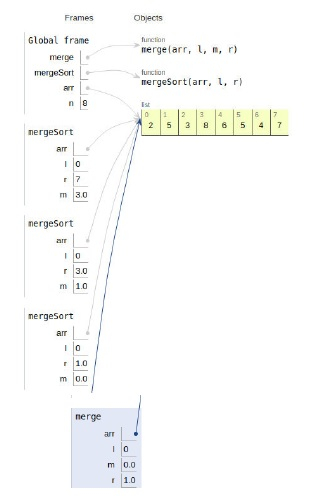# Python Program for Merge Sort

Problem statement − We are given an array, we need to sort it using the concept of merge sort

Here we place the maximum element at the end. This is repeated until the array is sorted.

Now let’s observe the solution in the implementation below −

## Example

#merge function
def merge(arr, l, m, r):
n1 = m - l + 1
n2 = r- m
# create arrays
L =  * (n1)
R =  * (n2)
# Copy data to arrays
for i in range(0 , n1):
L[i] = arr[l + i]
for j in range(0 , n2):
R[j] = arr[m + 1 + j]
i = 0 # first half of array
j = 0 # second half of array
k = l # merges two halves
while i < n1 and j < n2 :
if L[i] <= R[j]:
arr[k] = L[i]
i += 1
else:
arr[k] = R[j]
j += 1
k += 1
# copy the left out elements of left half
while i < n1:
arr[k] = L[i]
i += 1
k += 1
# copy the left out elements of right half
while j < n2:
arr[k] = R[j]
j += 1
k += 1
# sort
def mergeSort(arr,l,r):
if l < r:
# getting the average
m = (l+(r-1))/2
# Sort
mergeSort(arr, l, m)
mergeSort(arr, m+1, r)
merge(arr, l, m, r)
# main
arr = [2,5,3,8,6,5,4,7]
n = len(arr)
mergeSort(arr,0,n-1)
print ("Sorted array is")
for i in range(n):
print (arr[i],end=" ")

## Output

Sorted array is
2 3 4 5 5 6 7 8All the variables are declared in the local scope and their references are seen in the figure above.

## Conclusion

In this article, we have learned about how we can make a Python Program for Merge Sort